# M-PSK Demodulator Baseband

Demodulate PSK-modulated data

•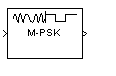Libraries:
Communications Toolbox / Modulation / Digital Baseband Modulation / PM
Communications Toolbox HDL Support / Modulation / PM

## Description

The M-PSK Demodulator Baseband block demodulates a baseband representation of a PSK-modulated signal. The modulation order, M, is equivalent to the number of points in the signal constellation and is determined by the M-ary number parameter. The block accepts scalar or column vector input signals.

## Examples

expand all

Use the Open model button to open the doc_8psk_model model. The model generates an 8-PSK signal, applies white noise, displays the resulting constellation diagram, and computes the error statistics.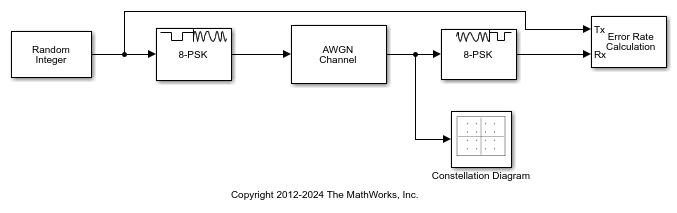Run the model.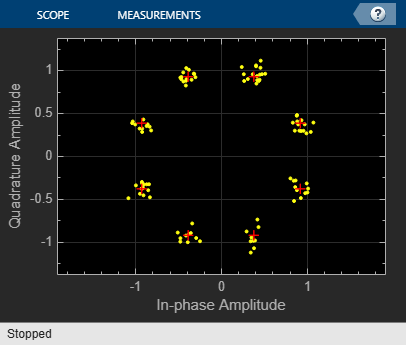The error statistics are collected in vector ErrorVec. Because Eb/No is 15 dB, there are no measured symbol errors.

Number of symbol errors = 0 

Change the Eb/No of the AWGN Channel block from 15 dB to 5 dB. The increase in the noise is shown in the constellation diagram.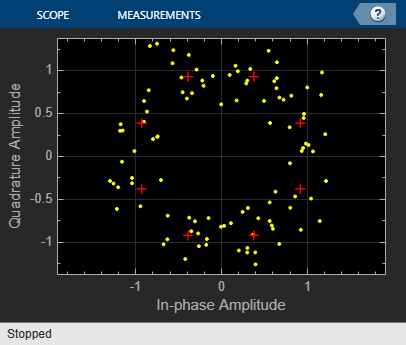Because of the increase in the noise level, the number of symbol errors is greater than zero.

Number of symbol errors = 21 

This example uses the doc_gray_code to compute bit error rates (BER) and symbol error rates (SER) for M-PSK modulation. The theoretical error rate performance of M-PSK modulation in AWGN is compared to the error rate performance for Gray-coded symbol mapping and to the error rate performance of binary-coded symbol mapping.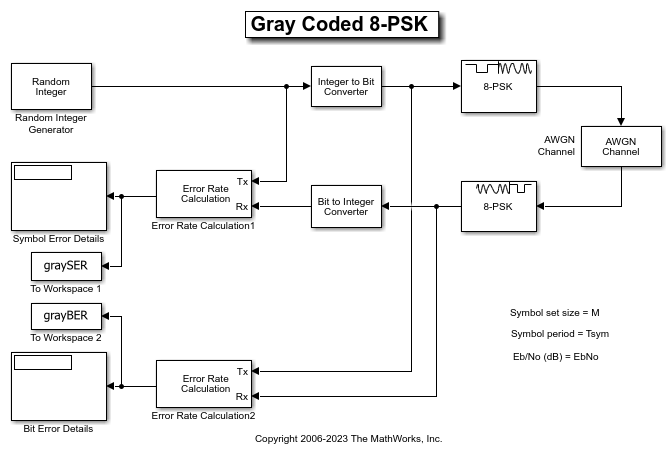The Random Integer Generator block serves as the source, producing a sequence of integers. The Integer to Bit Converter block converts each integer into a corresponding binary representation. The M-PSK Modulator Baseband block in the doc_gray_code model:

• Accepts binary-valued inputs that represent integers in the range [0, (M - 1], where M is the modulation order.

• Maps binary representations to constellation points using a Gray-coded ordering.

• Produces unit-magnitude complex phasor outputs, with evenly spaced phases in the range [0, (2(M - 1) / M)].

The AWGN Channel block adds white Gaussian noise to the modulated data. The M-PSK Demodulator Baseband block demodulates the noisy data. The Bit to Integer Converter block converts each binary representation to a corresponding integer. Then two separate Error Rate Calculation blocks calculate the error rates of the demodulated data. The block labeled SER Calculation compares the integer data to compute the symbol error rate statistics and the block labeled BER Calculation compares the bits data to compute the bit error rate statistics. The output of the Error Rate Calculation block is a three-element vector containing the calculated error rate, the number of errors observed, and the amount of data processed.

To reduce simulation run time and ensure that the statistics of the errors remain stable as the Eb/N0 ratio increases, the model is configured to run until 100 errors occur or until 1e8 bits have been transmitted.

The model initializes variables used to configure block parameters by using the PreLoadFcn callback function. For more information, see Model Callbacks (Simulink).

Produce Error Rate Curves

Compute the theoretical BER for nondifferential 8-PSK in AWGN over a range of Eb/N0 values by using the berawgn function. Simulate the doc_gray_code model with Gray-coded symbol mapping over the same range of Eb/N0 values.

Compare Gray coding with binary coding, by modifying the M-PSK Modulator Baseband and M-PSK Demodulator Baseband blocks to set the Constellation ordering parameter to Binary instead of Gray. Simulate the doc_gray_code model with binary-coded symbol mapping over the same range of Eb/N0 values.

Plot the results by using the semilogy function. The Gray-coded system achieves better error rate performance than the binary-coded system. Further, the Gray-coded error rate aligns with the theoretical error rate statistics.## Ports

### Input

expand all

Input port accepting a baseband representation of a PSK-modulated signal.

Data Types: single | double | int8 | int16 | int32 | uint8 | uint16 | uint32 | Boolean

### Output

expand all

Output signal, returned as a scalar or vector. The output is a demodulated version of the PSK-modulated signal.

Data Types: single | double | fixed point

## Parameters

expand all

To edit block parameters interactively, use the Property Inspector. From the Simulink® Toolstrip, on the Simulation tab, in the Prepare gallery, select .

Specify the modulation order as a positive integer power of two.

Example: 2 | 16

Specify the elements of the input signal as integers or bits. If Output type is Bit, the number of samples per frame is an integer multiple of the number of bits per symbol, log2(M).

Specify the demodulator output to be hard decision, log-likelihood ratio (LLR), or approximate LLR. The LLR and approximate LLR outputs are used with error decoders that support soft-decision inputs such as a Viterbi Decoder, to achieve superior performance. This parameter is available when Output type is Bit.

See Phase Modulation for algorithm details. The output values for Log-likelihood ratio and Approximate log-likelihood ratio decision types are of the same data type as the input values

Specify the source of the noise variance estimate. This parameter is available when Decision type is Log-likelihood ratio or Approximate log-likelihood ratio.

• To specify the noise variance from the dialog box, select Dialog.

• To input the noise variance from an input port, select Port.

Specify the estimate of the noise variance as a positive scalar. This parameter is available when Noise variance source is Dialog.

This parameter is tunable in all simulation modes. If you use the Simulink Coder™ rapid simulation (RSIM) target to build an RSIM executable, then you can tune the parameter without recompiling the model. Avoiding recompilation is useful for Monte Carlo simulations in which you run the simulation multiple times (perhaps on multiple computers) with different amounts of noise.

Note

The exact LLR algorithm computes exponentials using finite precision arithmetic. Computation of exponentials with very large positive or negative magnitudes might yield:

• Inf or -Inf if the noise variance is a very large value

• NaN if both the noise variance and signal power are very small values

When the output returns any of these values, try using the approximate LLR algorithm because it does not compute exponentials.

Specify how the integer or group of log2(M) bits is mapped to the corresponding symbol.

• When Constellation ordering is set to Gray, the output symbol is mapped to the input signal using a Gray-encoded signal constellation.

• When Constellation ordering is set to Binary, the modulated symbol is exp(+j2πm/M), where ϕ is the phase offset in radians, m is the integer output such that 0 ≤ m ≤ M – 1, and M is the modulation order.

• When Constellation ordering is User-defined, specify a vector of size M, which has unique integer values in the range [0, M–1]. The first element of this vector corresponds to the constellation point having a value of e with subsequent elements running counterclockwise.

Example: [0 3 2 1]

Specify the order in which input integers are mapped to output integers. The parameter is available when Constellation ordering is User-defined, and must be a row or column vector of size M having unique integer values in the range [0, M – 1].

The first element of this vector corresponds to the constellation point at 0 + Phase offset angle, with subsequent elements running counterclockwise. The last element corresponds to the -2π/M + Phase offset constellation point.

Specify, in radians, the phase offset of the initial constellation as a real scalar.

Example: pi/4

Specify the data type of the demodulated output signal.

The Data Type Assistant helps you set data attributes. To use the Data Type Assistant, click. For more information, see Specify Data Types Using Data Type Assistant (Simulink).

## Block Characteristics

 Data Types Boolean | double | fixed pointa, b, c | integer | single Multidimensional Signals no Variable-Size Signals yes a M = 2, 4, 8 only.b Fixed-point inputs must be signed.c When ASIC/FPGA is selected in the Hardware Implementation Pane, output is ufix(1) for bit outputs, and ufix(ceil(log2(M))) for integer outputs.

## Algorithms

expand all

 Proakis, John G. Digital Communications. 4th ed. New York: McGraw Hill, 2001.